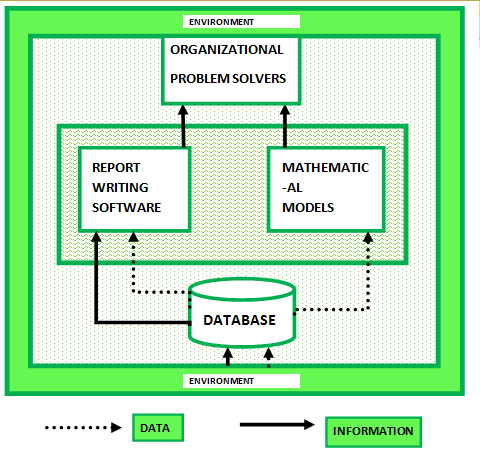# Management Information System (MIS) Models

A model is an abstraction of something it represents (some phenomenon), called an entity. For example, if a model represents a firm, then the firm is an entity.

Types of Models:

1. Physical Models:
It is a model that exists in three-dimensional form. For example, clay model of a new automobile developed by designers.
2. Narrative Models:
It is created by verbal or written description. Also, these can be created anywhere without much resources hence they are most popular.

3. Graphs:
It is usually a two-dimensional diagram. For example, graphs and charts.

4. Mathematical Models:
It uses symbolic notations and mathematical equations to represent a system. It can be represented by 3-D also. Following are the types of mathematical models:

• (a). Influence of Time:
Static Model does not include time as a variable whereas, Dynamic Model allows the changes of system attributes to be derived as a function of time.

• (b). Degree of Certainty:
A probability is a chance of occurring something will happen.Probabilities ranges from 0 to 1. A model that includes probabilities is called Probabilistic model, otherwise it is called a Deterministic model.

• (c). Ability to Achieve Optimization:
Optimizing Model selects the best solution among the alternatives.A SUB-OPTIMIZING MODEL permits the manager to enter a set of decisions, once this step is completed the model will project an outcome. It leaves the decision task on the manager.

Steps of Model Construction:

• Define the problem and the factor that influence it.
• Select criteria to guide the decision and establish objectives.
• Formulate a model that helps management to understand the relationships between the influence factors and the objectives the firm is trying to achieve.
• Collect the relevant data while trying to avoid the incorporation of superfluous information into the model.
• Identify and evaluate the alternatives.
• Select the best alternative.
• Implement the alternative.

The below shown figure is an model of Management Information System (MIS).Components of MIS Model:

1. Organizational Problem Solvers:
Outputs are used by persons who are responsible for solving the firm’s problems(say managers).

2. Database:
It’s contents are used by software that produces report as well as mathematical model.

3. Mathematical Model:
It produces information as a simulation of firm’s operation.

4. Report Writing Software:
It produces both periodic and special report.

5. Environment:
The environment is of great importance to the firm. It is the very reason of the firm’s existence.

• Can be a learning experience.
• Provides predictive power.
• Less expensive than trial and error method.
• Speed allows consideration of more options.